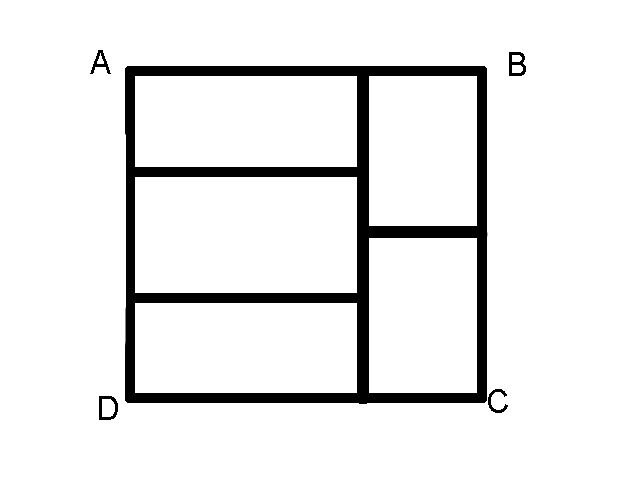# Combining 5 Rectangles

Geometry Level 2Rectangle ABCD (above) is made out of 5 rectangles of equal length and breadth. If the perimeter of ABCD is 44, what is the perimeter of one of these smaller rectangles?

×